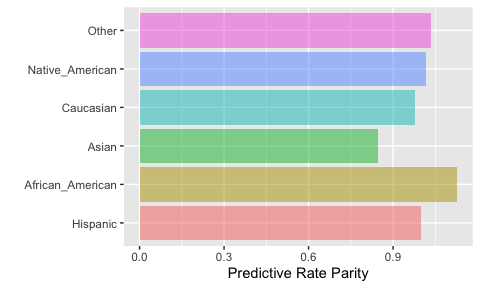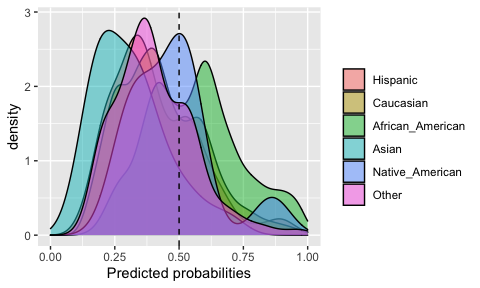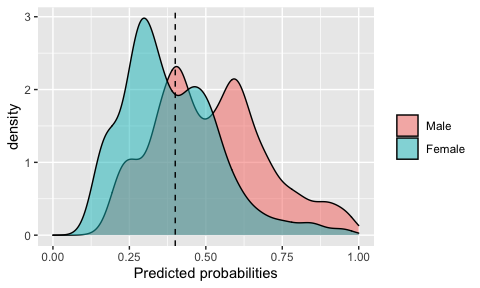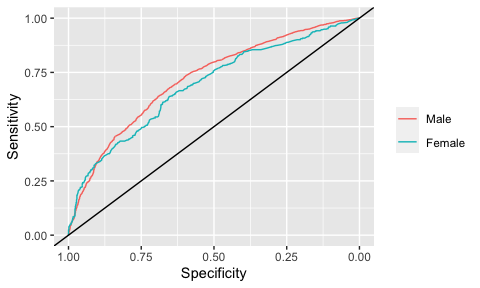Tutorial to the fairness R package

2021-04-14

This vignette provides a brief tutorial on the fairness R package. A more detailed tutorial is provided in this blogpost.

To date, a number of algorithmic fairness metrics have been proposed. Demographic parity, proportional parity and equalized odds are among the most commonly used metrics to evaluate fairness across sensitive groups in binary classification problems (with supervised machine learning algorithms). Multiple other metrics have been proposed based on performance measures extracted from the confusion matrix (e.g., false positive rate parity, false negative rate parity).

The fairness R package provides tools to easily calculate fairness metrics across different sensitive groups given predicted probabilities or predicted classes The package also provides visualizations that make it easier to comprehend these metrics and biases between subgroups of the data.

The package implements the following metrics and parities:

• Demographic parity
• Proportional parity
• Equalized odds
• Predictive rate parity
• False positive rate parity
• False negative rate parity
• Accuracy parity
• Negative predictive value parity
• Specificity parity
• ROC AUC parity
• MCC parity

Installation

Install the latest stable package version from CRAN:

install.packages('fairness')
library(fairness)

…or get the most recent development version from Github:

library(devtools)
devtools::install_github('kozodoi/fairness')
library(fairness)

Data description

This package includes two datasets to study algorithmic fairness: compas and germancredit. In this tutorial, you will be able to use a simplified version of the landmark COMPAS dataset. You can read more about the dataset here. To load the dataset, all you need to do is:

data('compas')

The compas dataframe contains nine columns: The outcome is Two_yr_Recidivism, i.e. whether an individual will commit a crime in two years or not. Variables exist in the data about prior criminal record (Number_of_Priors and Misdemeanor) and basic features such as age, categorized (Age_Above_FourtyFive and Age_Below_TwentyFive), sex (Female) and ethnicity (ethnicity). You don’t really need to delve into the data much, we have already ran a prediction model using all variables to predict Two_yr_Recidivism and concatenated the predicted probabilities (probability) and predicted classes (predicted) to the data. You will be able to use the probability and predicted columns directly in your analysis.

Please feel free to set up other prediction models (e.g. excluding sensitive group information, such as sex and ethnicity) and use your generated predicted probabilities or classes to assess group fairness.

An outlook on the confusion matrix

Most fairness metrics are calculated based on a confusion matrix produced by a classification model. The confusion matrix is comprised of four classes:

• True positives (TP): the true class is positive and the prediction is positive (correct classification)
• False positives (FP): the true class is negative and the prediction is positive (incorrect classification)
• True negatives (TN): the true class is negative and the prediction is negative (correct classification)
• False negatives (FN): the true class is positive and the prediction is negative (incorrect classification)

Fairness metrics are calculated by comparing one or more of these measures across sensitive subgroups (e.g., male and female). For a detailed overview of measures coming from the confusion matrix and precise definitions, click here or here.

Fairness metrics functions

The package implements 11 fairness metrics. Many of these are mutually exclusive: results for a given classification problem often cannot be fair in terms of all metrics. Depending on a context, it is important to select an appropriate metric to evaluate fairness.

Below, we describe functions used to compute the implemented metrics. Every function has a similar set of arguments:

• data: data.frame containing the input data and model predictions
• group: column name indicating the sensitive group (factor variable)
• base: base level of the sensitive group for fairness metrics calculation
• outcome: column name indicating the binary outcome variable
• outcome_base: base level of the outcome variable (i.e., negative class) for fairness metrics calculation

We also need to supply model predictions. Depending on the metric, we need to provide either probabilistic predictions as probs or class predictions as preds. The model predictions can be appended to the original data.frame or provided as a vector. In this tutorial, we will use probabilistic predictions with all functions. When working with probabilistic predictions, some metrics require a cutoff value to convert probabilities into class predictions supplied as cutoff.

Before looking at different metrics, we will create a binary numeric version of the outcome variable that we will supply as outcome in fairness metrics functions:

compas$Two_yr_Recidivism_01 <- ifelse(compas$Two_yr_Recidivism == 'yes', 1, 0)

Demographic parity

Demographic parity is one of the most popular fairness indicators in the literature. Demographic parity is achieved if the absolute number of positive predictions in the subgroups are close to each other. This measure does not take true class into consideration and only depends on the model predictions.

Formula: (TP + FP)

dem_parity(data    = compas,
outcome = 'Two_yr_Recidivism_01',
group   = 'ethnicity',
probs   = 'probability',
cutoff  = 0.5,
base    = 'Caucasian')

Proportional parity

Proportional parity is very similar to demographic parity but modifies it to address the issue discussed above. Proportional parity is achieved if the proportion of positive predictions in the subgroups are close to each other. Similar to the demographic parity, this measure also does not depend on the true labels.

Formula: (TP + FP) / (TP + FP + TN + FN)

prop_parity(data    = compas,
outcome = 'Two_yr_Recidivism_01',
group   = 'ethnicity',
probs   = 'probability',
cutoff  = 0.5,
base    = 'Caucasian')

All the rest of the functions take the true class into consideration.

Equalized odds

Equalized odds are achieved if the sensitivities in the subgroups are close to each other. The group-specific sensitivities indicate the number of the true positives divided by the total number of positives in that group.

Formula: TP / (TP + FN)

equal_odds(data    = compas,
outcome = 'Two_yr_Recidivism_01',
group   = 'ethnicity',
probs   = 'probability',
cutoff  = 0.5,
base    = 'African_American')

Predictive rate parity

Predictive rate parity is achieved if the precisions (or positive predictive values) in the subgroups are close to each other. The precision stands for the number of the true positives divided by the total number of examples predicted positive within a group.

Formula: TP / (TP + FP)

pred_rate_parity(data    = compas,
outcome = 'Two_yr_Recidivism_01',
group   = 'ethnicity',
probs   = 'probability',
cutoff  = 0.5,
base    = 'African_American')

Accuracy parity

Accuracy parity is achieved if the accuracies (all accurately classified examples divided by the total number of examples) in the subgroups are close to each other.

Formula: (TP + TN) / (TP + FP + TN + FN)

acc_parity(data    = compas,
outcome = 'Two_yr_Recidivism_01',
group   = 'ethnicity',
probs   = 'probability',
preds   = NULL,
cutoff  = 0.5,
base    = 'African_American')

False negative rate parity

False negative rate parity is achieved if the false negative rates (the ratio between the number of false negatives and the total number of positives) in the subgroups are close to each other.

Formula: FN / (TP + FN)

fnr_parity(data    = compas,
outcome = 'Two_yr_Recidivism_01',
group   = 'ethnicity',
probs   = 'probability',
cutoff  = 0.5,
base    = 'African_American')

False positive rate parity

False positive rate parity is achieved if the false positive rates (the ratio between the number of false positives and the total number of negatives) in the subgroups are close to each other.

Formula: FP / (TN + FP)

fpr_parity(data    = compas,
outcome = 'Two_yr_Recidivism_01',
group   = 'ethnicity',
probs   = 'probability',
cutoff  = 0.5,
base    = 'African_American')

Negative predictive value parity

Negative predictive value parity is achieved if the negative predictive values in the subgroups are close to each other. The negative predictive value is computed as a ratio between the number of true negatives and the total number of predicted negatives. This function can be considered the ‘inverse’ of the predictive rate parity.

Formula: TN / (TN + FN)

npv_parity(data    = compas,
outcome = 'Two_yr_Recidivism_01',
group   = 'ethnicity',
probs   = 'probability',
cutoff  = 0.5,
base    = 'African_American')

Specificity parity

Specificity parity is achieved if the specificities (the ratio of the number of the true negatives and the total number of negatives) in the subgroups are close to each other. This function can be considered the ‘inverse’ of the equalized odds.

Formula: TN / (TN + FP)

spec_parity(data    = compas,
outcome = 'Two_yr_Recidivism_01',
group   = 'ethnicity',
probs   = 'probability',
cutoff  = 0.5,
base    = 'African_American')

Two additional comparisons are implemented, namely ROC AUC and Matthews correlation coefficient comparisons.

ROC AUC comparison

This function calculates ROC AUC and visualizes ROC curves for all subgroups. Note that probabilities must be defined for this function. Also, as ROC evaluates all possible cutoffs, the cutoff argument is excluded from this function.

roc_parity(data    = compas,
outcome = 'Two_yr_Recidivism_01',
group   = 'ethnicity',
probs   = 'probability',
base    = 'African_American')

Matthews correlation coefficient comparison

The Matthews correlation coefficient (MCC) takes all four classes of the confusion matrix into consideration. MCC is sometimes referred to as the single most powerful metric in binary classification problems, especially for data with class imbalances.

Formula: (TP×TN-FP×FN)/√((TP+FP)×(TP+FN)×(TN+FP)×(TN+FN))

mcc_parity(data    = compas,
outcome = 'Two_yr_Recidivism_01',
group   = 'ethnicity',
probs   = 'probability',
cutoff  = 0.5,
base    = 'African_American')

Output and visualizations

All functions output results and matching barcharts that provide visual cues about the parity metrics for the defined sensitive subgroups. For instance, let’s look at predictive rate parity with ethnicity being set as the sensitive group and considering Caucasians as the ‘base’ group:

output$Metric #> Caucasian African_American Asian Hispanic #> Precision 0.577381 0.6652475 0.5000000 0.590604 #> Predictive Rate Parity 1.000000 1.1521812 0.8659794 1.022902 #> Group size 2103.000000 3175.0000000 31.0000000 509.000000 #> Native_American Other #> Precision 0.600000 0.6117647 #> Predictive Rate Parity 1.039175 1.0595512 #> Group size 11.000000 343.0000000 In the upper row, the raw precision values are shown for all ethnicities, and in the row below, the relative precisions compared to Caucasians (1) are shown. Note that in case an other ethnic group is set as the base group (e.g. Hispanic), the raw precision values do not change, only the relative metrics: output$Metric
#>                          Hispanic    Caucasian African_American      Asian
#> Precision                0.590604    0.5773810        0.6652475  0.5000000
#> Predictive Rate Parity   1.000000    0.9776109        1.1263849  0.8465909
#> Group size             509.000000 2103.0000000     3175.0000000 31.0000000
#>                        Native_American       Other
#> Precision                     0.600000   0.6117647
#> Predictive Rate Parity        1.015909   1.0358289
#> Group size                   11.000000 343.0000000

A standard output is a barchart that shows the relative metrics for all subgroups. For the previous case (when Hispanic is defined as the base group), this plot would look like this:

output$Metric_plotWhen probabilities are defined, an extra density plot will be output with the distributions of probabilities of all subgroups and the user-defined cutoff: output$Probability_plotAnother example would be comparing males vs. females in terms of recidivism prediction and defining a 0.4 cutoff:

output$Probability_plotThe function related to ROC AUC comparisons will output ROC curves for each subgroups. Let’s look at the plot, also comparing males vs. females: output$ROCAUC_plotClosing words

You have read through the fairness R package tutorial and by now, you have a solid grip on algorithmic group fairness metrics. If something is not clear, check out this blogpost with a more detailed tutorial. We hope that you will be able to use this R package in your data analysis! Please let us know if you have any issues here - fairness GitHub - or contact the authors if you have any feedback!